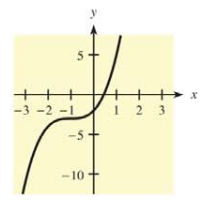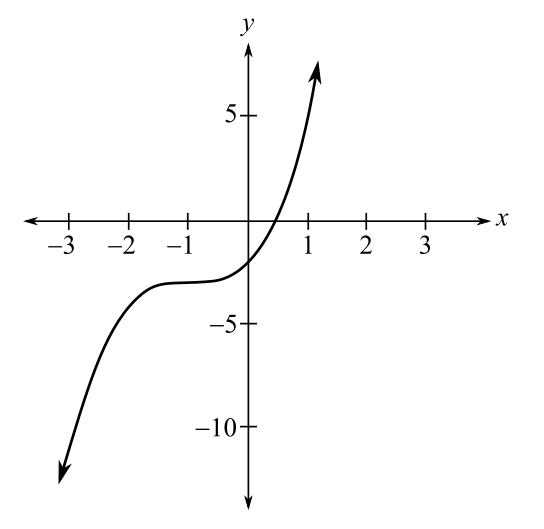Chapter 10.1, Problem 13E### Mathematical Applications for the ...

11th Edition
Ronald J. Harshbarger + 1 other
ISBN: 9781305108042

#### Solutions

Chapter
Section### Mathematical Applications for the ...

11th Edition
Ronald J. Harshbarger + 1 other
ISBN: 9781305108042
Textbook Problem

# For each function and graph in Problems 11-14(a) estimate the coordinates of the relative maxima, relative minima, or horizontal points of inflection by observing the graph.(b) use y' = f'(x) to find the critical values.(c) find the critical points.(d) Do the results in part (c) confirm your estimates in part (a)? y = x 3 + 3 x 2 + 3 x − 2(a)

To determine

The relative maxima, relative minima, or horizontal points of inflection from the graph of the function y=x3+3x2+3x2 and the graph of the function is shown below:Explanation

Given Information:

The provided function is, y=x3+3x2+3x2.

Explanation:

Consider the provided function y=x3+3x2+3x2.

The graph of the provided function is shown below,

The critical values are the values at which the graph can have turning points, the derivative cannot change sign anywhere except at the critical value.

From the above graph, there is no change in the sign of critical values as the graph only increases

(b)

To determine

To calculate: The critical values of the function y=x3+3x2+3x2 by using the derivative.

(c)

To determine

To calculate: The critical points of the function y=x3+3x2+3x2.

(d)

To determine

If the results in part (c) confirm the estimates in part (a).

### Still sussing out bartleby?

Check out a sample textbook solution.

See a sample solution

#### The Solution to Your Study Problems

Bartleby provides explanations to thousands of textbook problems written by our experts, many with advanced degrees!

Get Started

#### Find more solutions based on key concepts